# Balancing Chemical Equations. Balancing redox equationsPage 7

#### WATCH ALL SLIDES

0

-1

-3

0

-4

-1

0

Slide 28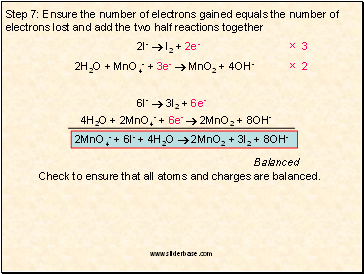Step 7: Ensure the number of electrons gained equals the number of electrons lost and add the two half reactions together

2I-  I2 + 2e-

× 3

2H2O + MnO4- + 3e-  MnO2 + 4OH-

× 2

6I-  3I2 + 6e-

4H2O + 2MnO4- + 6e-  2MnO2 + 8OH-

2MnO4- + 6I- + 4H2O  2MnO2 + 3I2 + 8OH-

Balanced

Check to ensure that all atoms and charges are balanced.

Slide 29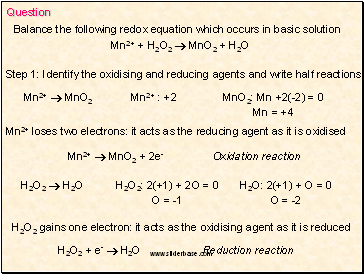Question

Balance the following redox equation which occurs in basic solution

Mn2+ + H2O2  MnO2 + H2O

Step 1: Identify the oxidising and reducing agents and write half reactions

Mn2+  MnO2

Mn2+ : +2

MnO2: Mn +2(-2) = 0

Mn = +4

Mn2+ loses two electrons: it acts as the reducing agent as it is oxidised

Mn2+  MnO2 + 2e- Oxidation reaction

H2O2  H2O

H2O2: 2(+1) + 2O = 0

O = -1

H2O: 2(+1) + O = 0

O = -2

H2O2 gains one electron: it acts as the oxidising agent as it is reduced

H2O2 + e-  H2O Reduction reaction

Slide 30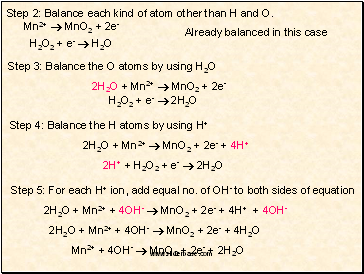Step 2: Balance each kind of atom other than H and O.

Step 3: Balance the O atoms by using H2O

2H2O + Mn2+  MnO2 + 2e-

H2O2 + e-  2H2O

Step 4: Balance the H atoms by using H+

2H2O + Mn2+  MnO2 + 2e- + 4H+

2H+ + H2O2 + e-  2H2O

Step 5: For each H+ ion, add equal no. of OH- to both sides of equation

2H2O + Mn2+ + 4OH-  MnO2 + 2e- + 4H+ + 4OH-

2H2O + Mn2+ + 4OH-  MnO2 + 2e- + 4H2O

Mn2+ + 4OH-  MnO2 + 2e- + 2H2O

Mn2+  MnO2 + 2e-

H2O2 + e-  H2O

Slide 31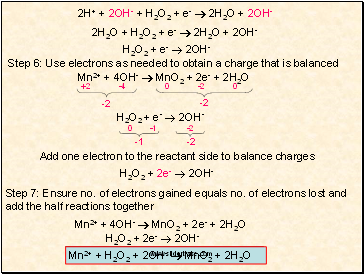2H+ + 2OH- + H2O2 + e-  2H2O + 2OH-

2H2O + H2O2 + e-  2H2O + 2OH-

H2O2 + e-  2OH-

Step 6: Use electrons as needed to obtain a charge that is balanced

Mn2+ + 4OH-  MnO2 + 2e- + 2H2O

-2

-2

H2O2 + e-  2OH-

-1

-2

Add one electron to the reactant side to balance charges

H2O2 + 2e-  2OH-

Step 7: Ensure no. of electrons gained equals no. of electrons lost and add the half reactions together

Mn2+ + 4OH-  MnO2 + 2e- + 2H2O

H2O2 + 2e-  2OH-

Mn2+ + H2O2 + 2OH-  MnO2 + 2H2O

+2

-4

0

-2

0

-2

-1

0

Slide 32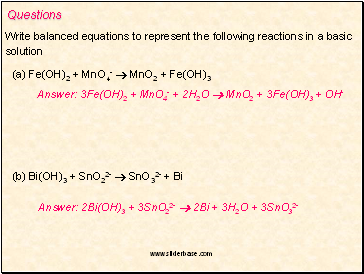Questions

Write balanced equations to represent the following reactions in a basic

solution

(a) Fe(OH)2 + MnO4-  MnO2 + Fe(OH)3

Go to page:
1  2  3  4  5  6  7  8